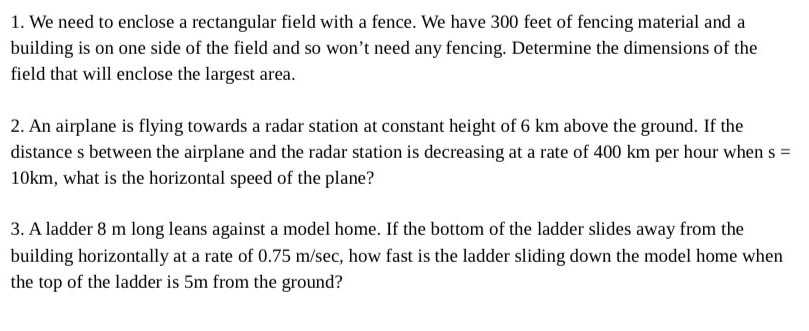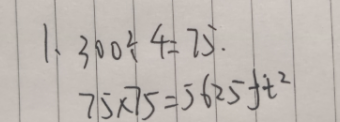### Still have math questions?

Arithmetic
Question1. We need to enclose a rectangular field with a fence. We have $$300$$ feet of fencing material and a building is on one side of the field and so won't need any fencing. Determine the dimensions of the field that will enclose the largest area.

2. An airplane is flying towards a radar station at constant height of $$6 km$$ above the ground. If the distance s between the airplane and the radar station is decreasing at a rate of $$400 km$$ per hour when $$s =$$ $$10 km$$ , what is the horizontal speed of the plane?

3. A ladder $$8 m$$ long leans against a model home. If the bottom of the ladder slides away from the building horizontally at a rate of $$0.75 m / sec$$ , how fast is the ladder sliding down the model home when the top of the ladder is $$5 m$$ from the ground?